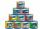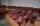# Digits

Show that if x, y, z are 3 consecutive nonzero digits, zyx-xyz = 198, where zyx and xyz are three-digit numbers created from x, y, z.

Result

a =  198

#### Solution:Leave us a comment of example and its solution (i.e. if it is still somewhat unclear...):

Showing 0 comments:Be the first to comment!## Next similar examples:

1. SequenceBetween numbers 1 and 53 insert n members of the arithmetic sequence that its sum is 702.
2. SeatsSeats in the sport hall are organized so that each subsequent row has five more seats. First has 10 seats. How many seats are: a) in the eighth row b) in the eighteenth row
3. CansHow many cans must be put in the bottom row if we want 182 cans arrange in 13 rows above so that each subsequent row has always been one tin less? How many cans will be in the top row?
4. Consecutive numbersSum of ten consecutive numbers is 105. Determine these numbers (write first and last).
5. Theorem proveWe want to prove the sentense: If the natural number n is divisible by six, then n is divisible by three. From what assumption we started?
6. Holidays - on poolChildren's tickets to the swimming pool stands x € for an adult is € 2 more expensive. There was m children in the swimming pool and adults three times less. How many euros make treasurer for pool entry?
7. TrucksThree lorries droved bricks. One drove n bricks at once, second m less bricks than the first and third 300 bricks more the first lorry. The first lorry went 4 times a day the largest went 3 times a day and the smallest 5 times a day. How many bricks br
8. Find the sumFind the sum of all natural numbers from 1 and 100, which are divisible by 2 or 5
9. ChairsDetermine the number of seats in the seventh row and ninth row, if 3rd row has 14 seats and in every next row of seats has five more than the previous row.
10. AS sequenceIn an arithmetic sequence is given the difference d = -3 and a71 = 455. a) Determine the value of a62 b) Determine the sum of 71 members.
11. Third memberDetermine the third member of the AP if a4=93, d=7.5.
12. Sequence 3Write the first 5 members of an arithmetic sequence: a4=-35, a11=-105.
13. Sequence 2Write the first 5 members of an arithmetic sequence a11=-14, d=-1
14. AP - simpleDetermine the first nine elements of sequence if a10 = -1 and d = 4
15. SequenceWrite the first 6 members of these sequence: a1 = 5 a2 = 7 an+2 = an+1 +2 an
16. SequenceWrite the first 7 members of an arithmetic sequence: a1=-3, d=6.
17. Fifth memberDetermine the fifth member of the arithmetic progression, if the sum of the second and fifth members equal to 73, and difference d = 7.